Next: Références Up: Annex: Variance of Sullivan Previous: Variance of

## Interest of variances of Health expectancies

Because estimates of Health expectanciesare means of random variables assumed to be independent, according to the central limit theorem, they tend to have normal distributions. So, the latter normal is often assumed. It permits to test if differences between two Sullivan indices are caused by random fluctuations or not.

Therefore, if it is wanted to test the hypothesis of equality of two health expectancies,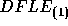and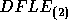(for example, for males and females, or two different times, countries...), it is sufficient to compute the following Z-score: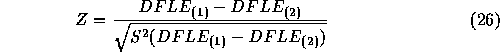If the absolute value of the statistic Z is too large (in comparison with what a normal law should give), the hypothesis is rejected. Then, the two health expectancies can be considered as not equal.

Since, we have always: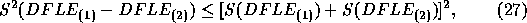where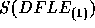and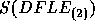are the standard errors ofand. Hence, the sum of the two standard errors (right hand of the previous equation) is used as denominator of equation 26. The level of significance for Z depends on the probability which is accepted for the case of a false conclusion of inequality.

For example, for p=0.05, the hypothesis is refused for:In general, see normal law distribution. Table 4 gives an example of such calculation of ratio Z. The differences between the two indicators DFLE does not seem to be the consequences of random fluctuations.

 AgeZ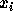1991 1981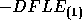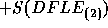0 70.11 0.25 67.77 0.23 2.34 0.48 4.86 *** 5 65.69 0.25 63.48 0.23 2.30 0.48 4.54 ***65 12.28 0.22 9.88 0.21 3.40 0.42 5.67 *** 70 8.39 0.21 6.86 0.20 1.93 0.41 4.73 *** 75 5.83 0.20 4.25 0.18 1.04 0.38 4.17 *** 80 3.39 0.18 2.34 0.16 1.96 0.34 3.04 *** 85 1.75 0.17 1.51 0.15 1.48 0.32 0.69 *** ***= probability of equality of the two indicators < 0.001.Next: Références Up: Annex: Variance of Sullivan Previous: Variance of

Eric Hauet
Fri Apr 25 22:40:35 DFT 1997